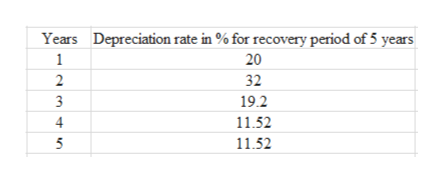A flour mill is considering buying a new jumbo sifter, which would have an installed cost of \$80,000. The new one would replace the existing sifter that was purchased 3 years ago at an installed cost of \$60,000. If the company moves forward with the replacement, it could sell the old sifter for \$19,000. Purchasing the new sifter would result in the company's current assets increasing by \$10,000 and current liabilities increasing by \$8,000. The company uses the 5-year MACRS table for depreciation and is taxed at 21%.a) What is the accumulated depreciation of the old sifter?b) What is the current book value of the old sifter?c) What is the amount of depreciation recapture/recovery?d) What is the tax on the sale of the old sifter?e) What are the after-tax proceeds from the sale of the old sifter?f) What is the change in Net Working Capital?g) What is the initial investment for the project?Asnwer e) - g). Asnwer in Excel

Question

A flour mill is considering buying a new jumbo sifter, which would have an installed cost of \$80,000. The new one would replace the existing sifter that was purchased 3 years ago at an installed cost of \$60,000. If the company moves forward with the replacement, it could sell the old sifter for \$19,000. Purchasing the new sifter would result in the company's current assets increasing by \$10,000 and current liabilities increasing by \$8,000. The company uses the 5-year MACRS table for depreciation and is taxed at 21%.

a) What is the accumulated depreciation of the old sifter?

b) What is the current book value of the old sifter?

c) What is the amount of depreciation recapture/recovery?

d) What is the tax on the sale of the old sifter?

e) What are the after-tax proceeds from the sale of the old sifter?

f) What is the change in Net Working Capital?

g) What is the initial investment for the project?

Asnwer e) - g). Asnwer in Excel

Step 1

Since we only answer up to 3 sub-parts, we’ll answer the first 3. Please resubmit the question and specify the other subparts if you’d like to get answer for other sub-parts as well.

Step 2

Modified Accelerated Cost Recovery (MACR) is a depreciation system by which assets are assigned with specific asset category for a designated depreciation time. The value of depreciation using MACRS method is lower than the straight line depreciation method. MACR table is used under three depreciation conventions such as half-year, mid-quarter and mid-month.

Step 3

(a)

Given:

Total cost (old sifter) = \$60,000

Useful life= 5 years

Assume that the asset has been purchased during the mid of the year. For this 200% declining depreciation balance is considered. Following table shows the ...help_outlineImage TranscriptioncloseDepreciation rate in % for recovery period of 5 years 20 Years 1 32 2 19.2 3 11.52 11.52 fullscreen

Want to see the full answer?

See Solution

Want to see this answer and more?

Our solutions are written by experts, many with advanced degrees, and available 24/7

See Solution
Tagged in